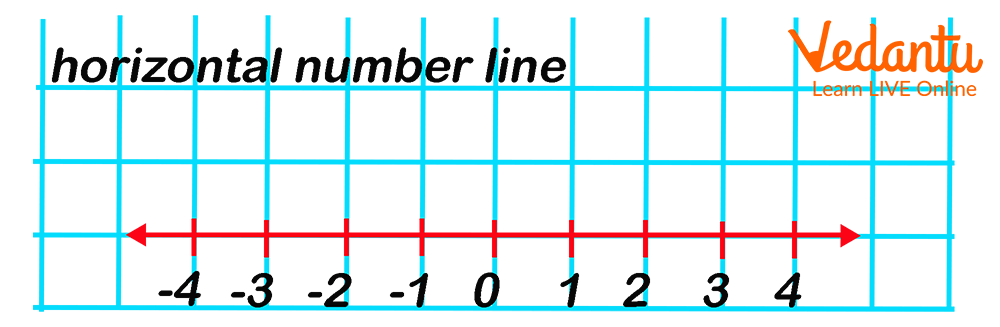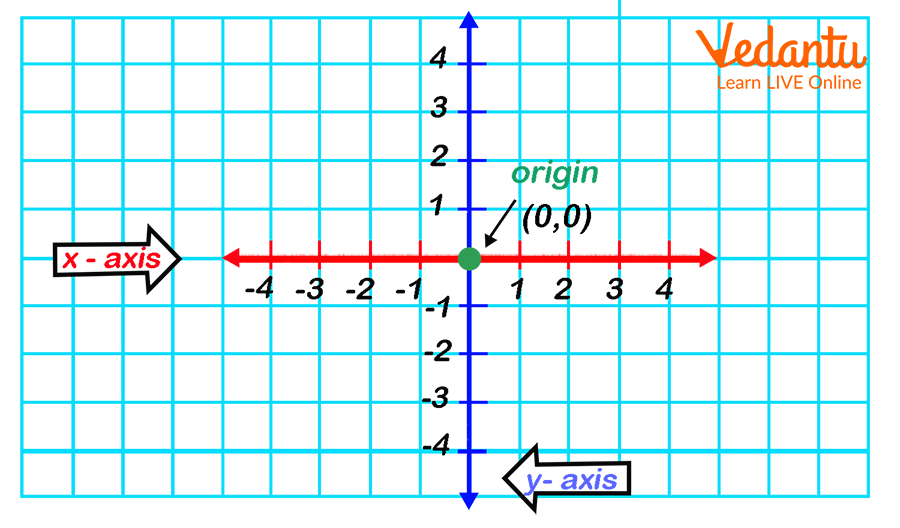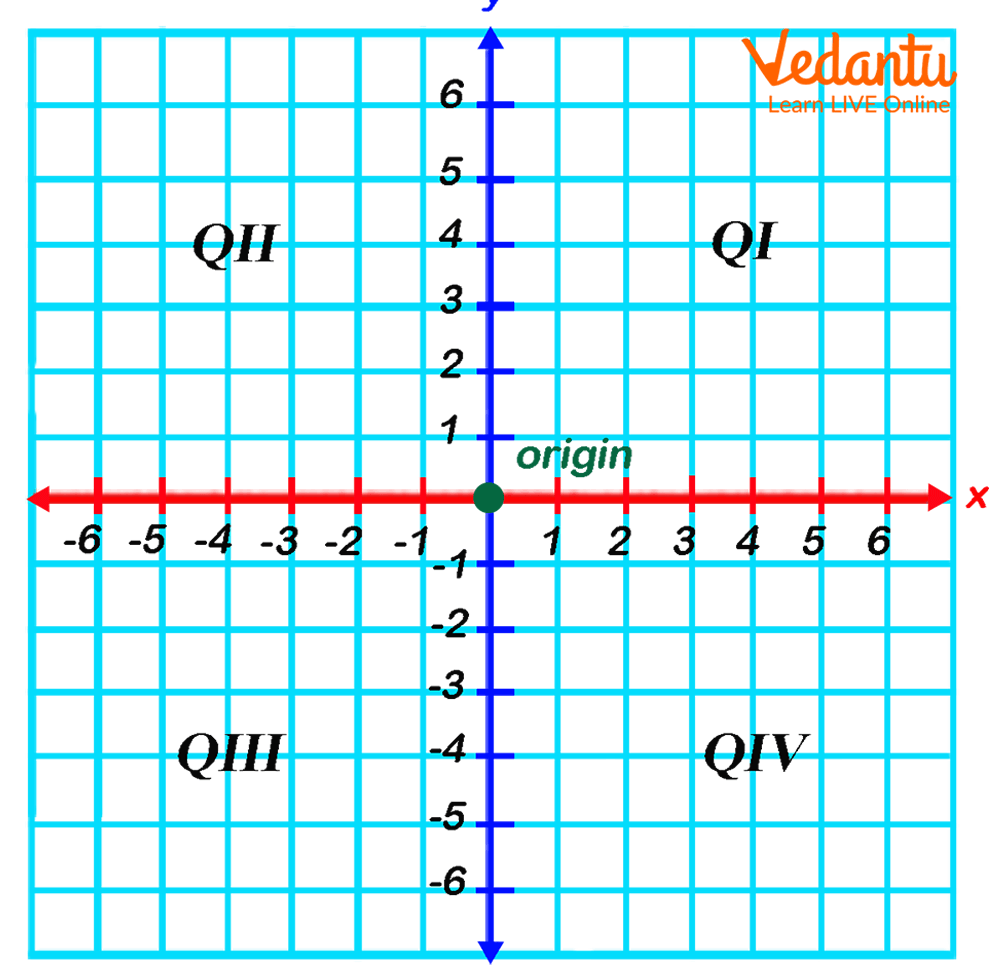Courses
Courses for Kids
Free study material
Free LIVE classes
More

# Y-Axis: Is This a Vertical Number Line?LIVE
Join Vedantu’s FREE Mastercalss

## Introduction to 2D Coordinate Geometry

We are all aware that the things in our environment are three-dimensional. Many shapes may be found in two dimensions as well. But do we know that specific knowledge of axes and planes can be used to depict these things on paper? We draw shapes in a 2D plane, which means we only have two directions to work with: x and y. What is the point of depicting the shapes in this manner? The coordinate plane concept is used by sailors to track their way back and construct maps. That way, you'll be able to find the object in no time. Do you want to know what coordinates, axes and their importance? Then, stay in this article till the end and learn more.

X and Y Coordinates can be used to describe the location of anything on a treasure map. The X and Y coordinates are two numbers that indicate how something on a flat surface is positioned. Flat objects like treasure maps are referred to as two-dimensional, or 2D, in mathematics. This is due to the fact that describing where something is on a two-dimensional surface requires only two numbers. Let's talk about Coordinates before we get into our topic, the Y-Axis.

## What are Coordinates?

We'll discuss Coordinates by looking at a number line.Horizontal Number Line

This is a number line with positive and negative values as well as integers. Because of the way it lays across, this is known as a horizontal number line. This is similar to the sun rising over the horizon. That is horizontal. The vertical number line is the next type of number line we'll discuss.Vertical Number Line

The above number line resembles a thermometer, right?

What would happen if we combined both of these number lines? This is something entirely different, and it's known as the coordinate plane.Combined Number Lines

On our coordinate plane, the red line is no longer referred to as a horizontal number line; instead, it is referred to as the x-axis. And the blue number line is now the y-axis, instead of just a vertical number line. The origin is a point in the center of the graph where both the x- and y-axis intersect. The coordinates of this origin are also known (0,0). So we are always going to start everything from the origin.

## Y-Axis

Assume your class is on the third floor of the school. The elevator can transport you to any floor. In fact, by observing where you decided to exit the elevator, someone could conclude which floor your class is on. Your class is at level 3 in the vertical direction. In mathematics, the vertical direction is usually denoted by the letter y. As a result, your class is at y = 3.

Assume the third floor has a single long hallway that runs east to west. One way to describe your location on the third floor is to say you're six classrooms east of the elevator. Someone who worked on the elevator would describe their location as "zero distance from the elevator." When measuring east-west, the elevator is the zero point.

As a result, when graphing on a Cartesian plane, the vertical orienting line is referred to as the y-axis. It tells you how high or low you are, just like an elevator. However, the y-axis, like the elevator, is the point from which you measure your horizontal location (right-left or east-west).

When these two axes intersect, they divide the coordinate plane into 4 segments. These four divisions are referred to as quadrants. As a result, we can say that the entire coordinate system is made up of four quadrants. The top right section is referred to as the first quadrant. The top left quadrant is referred to as the second quadrant. The bottom left quadrant is the third, and the bottom right quadrant is the fourth.4 Division

## Conclusion

Coordinates allow us to locate an object anywhere in the world. A coordinate plane is used to represent these coordinates. A coordinate plane is a two-dimensional plane with vertical and horizontal axes. The horizontal axis is referred to as the x-axis, and the vertical axis is referred to as the y-axis. We have discussed and understood about Y-Axis in this article.

Last updated date: 16th Sep 2023
Total views: 130.2k
Views today: 1.30k

## FAQs on Y-Axis: Is This a Vertical Number Line?

1. What makes a coordinate plane?

A coordinate plane is made up of axes, the origin, and quadrants. Axes are the two lines, vertical and horizontal, that never end in a coordinate plane. The horizontal axis is known as the x-axis or abscissa, while the vertical axis is known as the y-axis or ordinate. These axes intersect at a point known as the origin. The origin of any coordinate plane is its starting point.

2. What are coordinate systems designed for?

They are used to indicate a point's position on a plane or in space. A point in the plane can be uniquely represented in the Cartesian coordinate system by an ordered pair of numbers, each of which indicates a distance along an axis, measured from the origin.